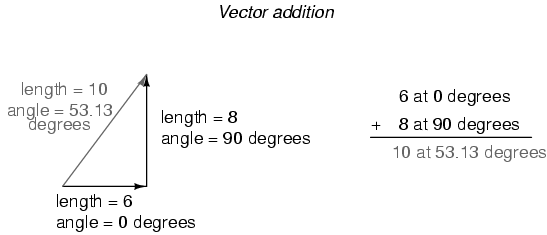2.4: Complex Vector Addition

If vectors with uncommon angles are added, their magnitudes (lengths) add up quite differently than that of scalar magnitudes: (Figure below)Vector magnitudes do not directly add for unequal angles.

If two AC voltages—90o out of phase—are added together by being connected in series, their voltage magnitudes do not directly add or subtract as with scalar voltages in DC. Instead, these voltage quantities are complex quantities, and just like the above vectors, which add up in a trigonometric fashion, a 6 volt source at 0o added to an 8 volt source at 90o results in 10 volts at a phase angle of 53.13o: (Figure below)The 6V and 8V sources add to 10V with the help of trigonometry.

Compared to DC circuit analysis, this is very strange indeed. Note that it is possible to obtain voltmeter indications of 6 and 8 volts, respectively, across the two AC voltage sources, yet only read 10 volts for a total voltage!

There is no suitable DC analogy for what we’re seeing here with two AC voltages slightly out of phase. DC voltages can only directly aid or directly oppose, with nothing in between. With AC, two voltages can be aiding or opposing one another to any degree between fully-aiding and fully-opposing, inclusive. Without the use of vector (complex number) notation to describe AC quantities, it would be very difficult to perform mathematical calculations for AC circuit analysis.

In the next section, we’ll learn how to represent vector quantities in symbolic rather than graphical form. Vector and triangle diagrams suffice to illustrate the general concept, but more precise methods of symbolism must be used if any serious calculations are to be performed on these quantities.

• REVIEW:

DC voltages can only either directly aid or directly oppose each other when connected in series. AC voltages may aid or oppose to any degree depending on the phase shift between them.